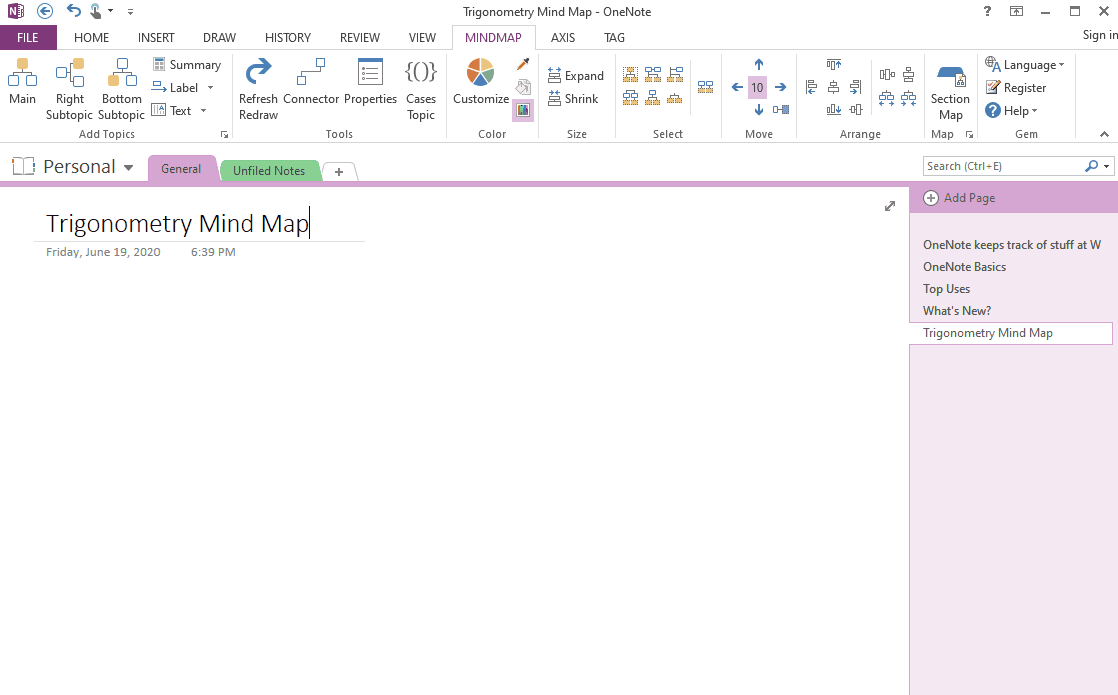### EXE: Use OneNote native Mathematical Equations in Mind Map

Because the Mind Map for OneNote uses OneNote's native objects, its topics can insert OneNote-native links, tables, tags, and even mathematical equations.

In this article, we'll show you how to apply OneNote's native mathematical equation to the topic of the mind map.

## Insert the Topic and Sub-topic in Mind Map

In the Mind Map tab:
1. Click “Main” button to create a new mind map.
2. Place the cursor in "Main Topic" and click on the "Right Subtopic" button to add a sub-topic.
3. Place the cursor in this sub-topic, click “Right Subtopic” again to add a new sub-topic for it.

## Apply Mathematical Equations in Topics

Place the cursor in a topic, click the Equation button (or press the shortcut Alt+=) under OneNote's Insert tab to insert the mathematical equation and start editing it.
At this point, a new Equation Tools / Design tab appears in the OneNote ribbon.

Equation input (<space> indicates pressing the space key):

Fractions use /, Example: a/b<space>
Vectors use /vec, Example: a/vec<space><space>

More equation input: OneNote Linear Format Equations## Demonstration